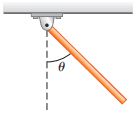# Dynamics of Rotational Motion and hinge

• denny2

#### denny2

One end of a thin, uniform rod is connected to a frictionless hinge as shown in Figure 1. The rod has a length of 0.8 mand a mass of 2 kg. It is held up in the horizontal position (θ=90∘) and then released.
1)Calculate the angular velocity of the rod at θ=90∘.
2)Calculate the angular acceleration of the rod at θ=90∘.
3)Calculate the angular velocity of the rod at θ=60∘
4)Calculate the angular acceleration of the rod at θ=60∘
5)Calculate the angular velocity of the rod at θ=0∘.
6)Calculate the angular acceleration of the rod at θ=0∘.## Homework Equations

mgh=1/2 Iw^2
a = ((mgcos)1/2)/I

## The Attempt at a Solution

I got these answers and only 1 and 6 were correct

Any help is appreciated thank you :)

Calculate the angular acceleration of the rod at θ=90∘.
3)Calculate the angular velocity of the rod at θ=60∘
4)Calculate the angular acceleration of the rod at θ=60∘
5)Calculate the angular velocity of the rod at θ=0∘.

you are having problem with above - so check expression for angular acceleration.
moment of inertia xangular acceleration = torque on the rod =forcex the the perpendicular distance from the axis of rotation
and for angular velocity one can use the work- energy conversion
work done by a torque is torquex angular displacement= rotational energy

•denny2
One end of a thin, uniform rod is connected to a frictionless hinge as shown in Figure 1. The rod has a length of 0.8 mand a mass of 2 kg. It is held up in the horizontal position (θ=90∘) and then released.
1)Calculate the angular velocity of the rod at θ=90∘.
2)Calculate the angular acceleration of the rod at θ=90∘.
3)Calculate the angular velocity of the rod at θ=60∘
4)Calculate the angular acceleration of the rod at θ=60∘
5)Calculate the angular velocity of the rod at θ=0∘.
6)Calculate the angular acceleration of the rod at θ=0∘.## Homework Equations

mgh=1/2 Iw^2
a = ((mgcos)1/2)/I

## The Attempt at a Solution

I got these answers and only 1 and 6 were correct